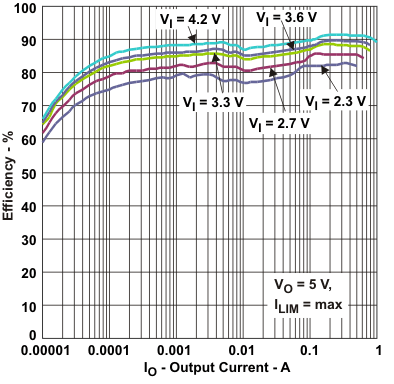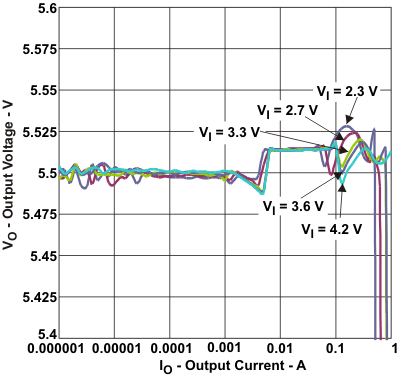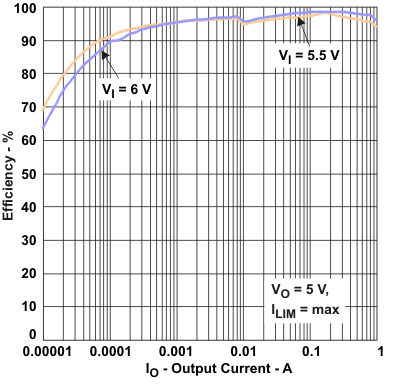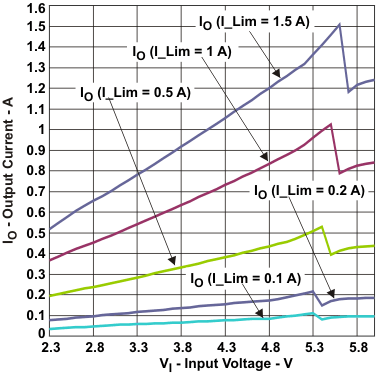SLVSAF7C September   2010  – April 2019

PRODUCTION DATA.

1. Features
2. Applications
3. Description
1.     Device Images
4. Revision History
5. Description (Continued)
6. Device Options
7. Pin Configuration and Functions
8. Specifications
9. Detailed Description
1. 9.1 Overview
2. 9.2 Functional Block Diagram
3. 9.3 Feature Description
4. 9.4 Device Functional Modes
10. 10Application and Implementation
1. 10.1 Application Information
2. 10.2 Typical Application
1. 10.2.1 Design Requirements
2. 10.2.2 Detailed Design Procedure
3. 10.2.3 Application Curves
11. 11Power Supply Recommendations
12. 12Layout
13. 13Device and Documentation Support
14. 14Mechanical, Packaging, and Orderable Information

• DSG|8
• DSG|8

Table 1. Table Of Graphs

DESCRIPTION FIGURE
Efficiency vs Output current (VOUT = 5.5 V, ILIM = 1.5 A, R1 = 2320 kΩ and R2 = 649 kΩ) Figure 1
vs Output current in 100% Duty-Cycle Mode (VOUT = 5.5V, ILIM = 1.5 A, R1 = 2320 kΩ and R2 = 649 kΩ) Figure 2
vs Input voltage (VOUT = 5.5 V, ILOAD = \{0.01;0.1; 1.0; 10; 100; 500 mA\}, R1 = 2320 kΩ and R2 = 649 kΩ) Figure 3
Maximum output current vs Input voltage (VOUT = 5.5 V, ILIM = \{100; 200; 500; 1000; 1500 mA\}, R1 = 1000 kΩ and R2 = 280 kΩ) Figure 4
Output voltage vs Output current (VOUT = 5.5 V, ILIM = 1.5 A, R1 = 1000 kΩ and R2 = 280 kΩ) Figure 5Figure 1. Efficiency vs Output CurrentFigure 3. Efficiency vs Input VoltageFigure 5. Output Voltage vs Output CurrentFigure 2. Efficiency vs Output Current In 100% Duty-Cycle ModeFigure 4. Maximum Output Current vs Input Voltage